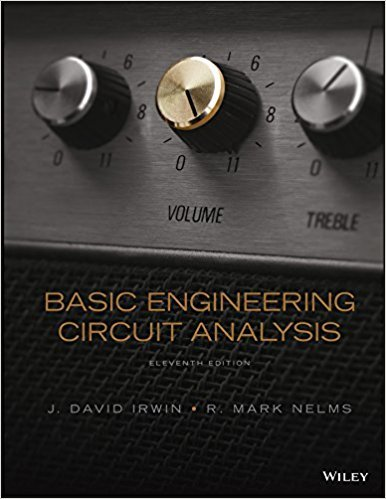×
Get Full Access to Basic Engineering Circuit Analysis - 11 Edition - Chapter 2 - Problem 2.71
Get Full Access to Basic Engineering Circuit Analysis - 11 Edition - Chapter 2 - Problem 2.71

×

# Answer: Determine the total resistance, RT, in the circuitISBN: 9781118539293 159

## Solution for problem 2.71 Chapter 2

Basic Engineering Circuit Analysis | 11th Edition

• Textbook Solutions
• 2901 Step-by-step solutions solved by professors and subject experts
• Get 24/7 help from StudySoup virtual teaching assistantsBasic Engineering Circuit Analysis | 11th Edition

4 5 1 349 Reviews
20
0
Problem 2.71

Determine the total resistance, RT, in the circuit in Fig. P2.71. Figure P2.71

Step-by-Step Solution:
Step 1 of 3

Post Lab 7: BJT Common­Emitter Circuit Voltage Gain Proposal: This experiment is designed to illustrate how the voltage gain of a CE bipolar transistor circuit can be changed by changing the bias of the circuit and by changing the values of the resistors in the circuit. Experimental Procedure: For the experiment you will require:  1 NPN transistor, either 2N3904, 2N2222, or equivalent  1 Curve tracer  1 Decade resistance box  NI-ELVIS workstation  Resistors and capacitors as noted in the schematic.  Linear graph paper or graphing software (e.g., Excel or MatLab). For part I: Establishing the proper Q-point (1),Use the curve tracer and measure the β of the transistor at a collector current of about 1.0mA and VCE of about 5 V. Set I MAX= 20 ma, V MAX= 10V,

Step 2 of 3

Step 3 of 3

##### ISBN: 9781118539293

Unlock Textbook Solution